panda python 可视化_python panda - CSDN

• 我的机器学习教程「美团」算法工程师带你入门机器学习 已经开始更新了，欢迎大家订阅~ 任何关于算法、编程、AI行业知识或博客内容的问题，可以随时扫码关注公众号「图灵的猫」，加入”学习小组“，沙雕博主在线答疑...
我的机器学习教程「美团」算法工程师带你入门机器学习   已经开始更新了，欢迎大家订阅~

任何关于算法、编程、AI行业知识或博客内容的问题，可以随时扫码关注公众号「图灵的猫」，加入”学习小组“，沙雕博主在线答疑~此外，公众号内还有更多AI、算法、编程和大数据知识分享，以及免费的SSR节点和学习资料。其他平台（知乎/B站）也是同名「图灵的猫」，不要迷路哦~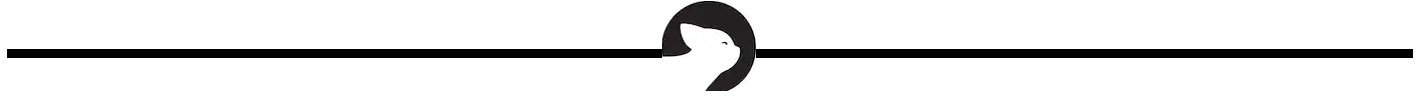大家都知道，Matplotlib 是众多 Python 可视化包的鼻祖，也是Python最常用的标准可视化库，其功能非常强大，同时也非常复杂，想要搞明白并非易事。但自从Python进入3.0时代以后，pandas的使用变得更加普及，它的身影经常见于市场分析、爬虫、金融分析以及科学计算中。

作为数据分析工具的集大成者，pandas作者曾说，pandas中的可视化功能比plt更加简便和功能强大。实际上，如果是对图表细节有极高要求，那么建议大家使用matplotlib通过底层图表模块进行编码。当然，我们大部分人在工作中是不会有这样变态的要求的，所以一句import pandas as pd就足够应付全部的可视化工作了。下面，我们总结一下PD库的一些使用方法和入门技巧。

一、线型图

对于pandas的内置数据类型，Series 和 DataFrame 都有一个用于生成各类 图表 的 plot 方法。 默认情况下， 它们所生成的是线型图。其实Series和DataFrame上的这个功能只是使用matplotlib库的plot()方法的简单包装实现。参考以下示例代码 -


import pandas as pd
import numpy as np

df = pd.DataFrame(np.random.randn(10,4),index=pd.date_range('2018/12/18',
periods=10), columns=list('ABCD'))

df.plot()


Python

执行上面示例代码，得到以下结果 -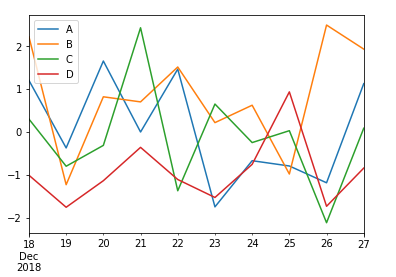如果索引由日期组成，则调用gct().autofmt_xdate()来格式化x轴，如上图所示。

我们可以使用x和y关键字绘制一列与另一列。

s = Series( np. random. randn( 10). cumsum(), index= np. arange( 0, 100, 10))
s. plot()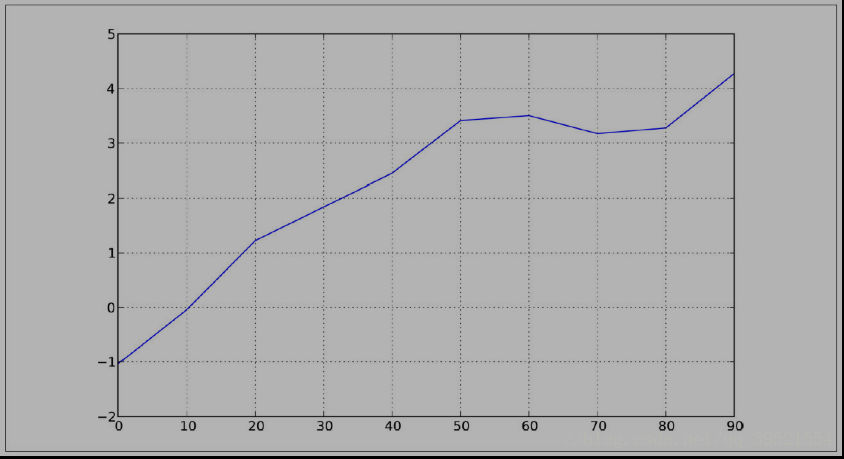pandas 的大部分绘图方法都有 一个 可选的ax参数， 它可以是一个 matplotlib 的 subplot 对象。 这使你能够在网格 布局 中 更为灵活地处理 subplot 的位置。 DataFrame的plot 方法会在 一个 subplot 中为各列绘制 一条 线， 并自动创建图例（ 如图所示）：

df = DataFrame( np. random. randn( 10, 4). cumsum( 0), ...: columns=[' A', 'B', 'C', 'D'], index= np. arange( 0, 100, 10))

df. plot()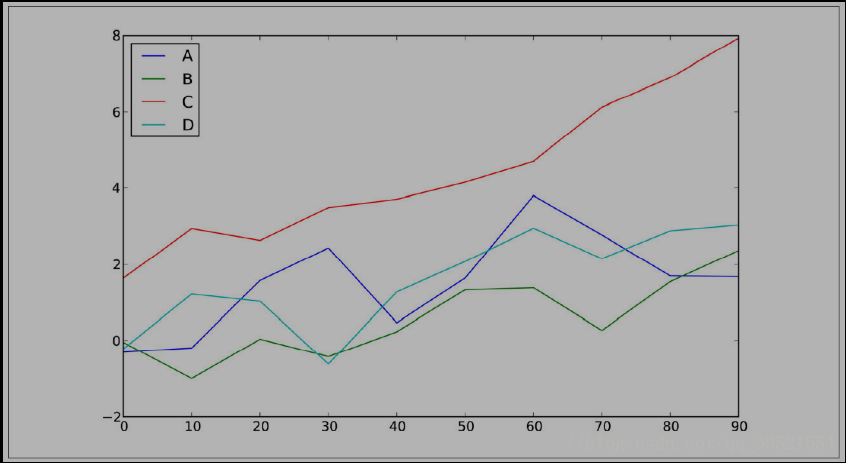二、柱状图

在生成线型图的代码中加上 kind=' bar'（ 垂直柱状图） 或 kind=' barh'（ 水平柱状图） 即可生成柱状图。 这时，Series 和 DataFrame 的索引将会被用 作 X（ bar） 或 （barh）刻度：

In : fig, axes = plt. subplots( 2, 1)

In : data = Series( np. random. rand( 16), index= list(' abcdefghijklmnop'))

In : data. plot( kind=' bar', ax= axes[ 0], color=' k', alpha= 0. 7)

Out[ 61]: < matplotlib. axes. AxesSubplot at 0x4ee7750>

In : data. plot( kind=' barh', ax= axes[ 1], color=' k', alpha= 0.

对于 DataFrame， 柱状 图 会 将 每一 行的 值 分为 一组， 如图 8- 16 所示：

In : df = DataFrame( np. random. rand( 6, 4), ...: index=[' one', 'two', 'three', 'four', 'five', 'six'], ...: columns= pd. Index([' A', 'B', 'C', 'D'], name=' Genus'))

In : df

Out[ 64]:

Genus

A         B         C         D
one 0. 301686 0. 156333 0. 371943 0. 270731
two 0. 750589 0. 525587 0. 689429 0. 358974
three 0. 381504 0. 667707 0. 473772 0. 632528
four 0. 942408 0. 180186 0. 708284 0. 641783
five 0. 840278 0. 909589 0. 010041 0. 653207
six 0. 062854 0. 589813 0. 811318 0. 060217

In : df. plot( kind=' bar')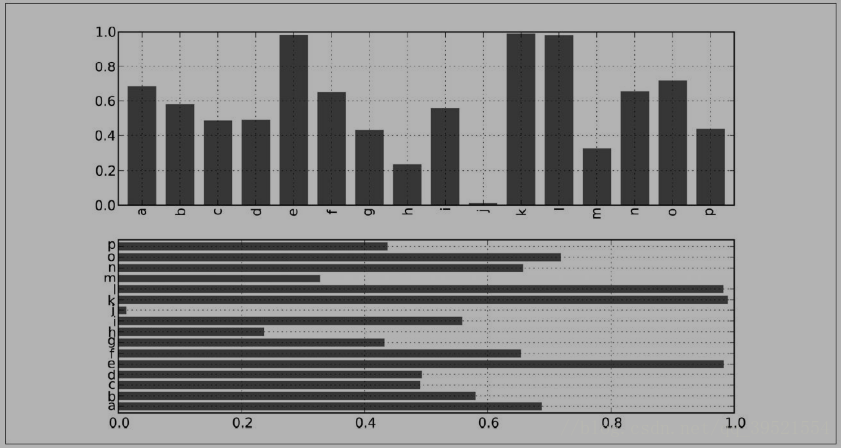三、条形图

现在通过创建一个条形图来看看条形图是什么。条形图可以通过以下方式来创建 -


import pandas as pd
import numpy as np

df = pd.DataFrame(np.random.rand(10,4),columns=['a','b','c','d'])
df.plot.bar()


Python

执行上面示例代码，得到以下结果 -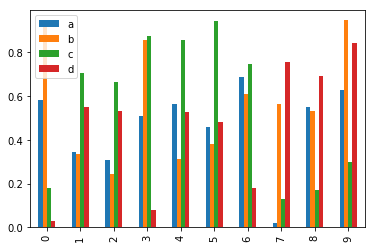要生成一个堆积条形图，通过指定：pass stacked=True -


import pandas as pd
df = pd.DataFrame(np.random.rand(10,4),columns=['a','b','c','d'])
df.plot.bar(stacked=True)


Python

执行上面示例代码，得到以下结果 -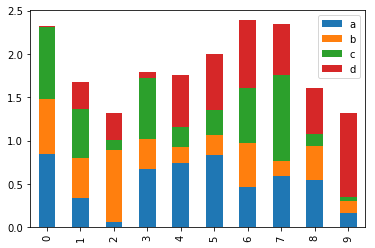要获得水平条形图，使用barh()方法 -


import pandas as pd
import numpy as np

df = pd.DataFrame(np.random.rand(10,4),columns=['a','b','c','d'])

df.plot.barh(stacked=True)


P

四、直方图

可以使用plot.hist()方法绘制直方图。我们可以指定bins的数量值。


import pandas as pd
import numpy as np

df = pd.DataFrame({'a':np.random.randn(1000)+1,'b':np.random.randn(1000),'c':
np.random.randn(1000) - 1}, columns=['a', 'b', 'c'])

df.plot.hist(bins=20)


Python

执行上面示例代码，得到以下结果 -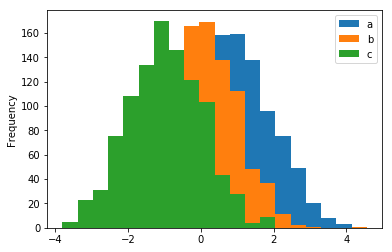要为每列绘制不同的直方图，请使用以下代码 -


import pandas as pd
import numpy as np

df=pd.DataFrame({'a':np.random.randn(1000)+1,'b':np.random.randn(1000),'c':
np.random.randn(1000) - 1}, columns=['a', 'b', 'c'])

df.hist(bins=20)


Python

执行上面示例代码，得到以下结果 -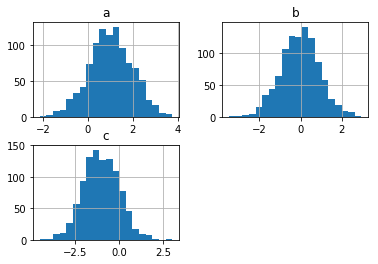五、箱型图

Boxplot可以绘制调用Series.box.plot()和DataFrame.box.plot()或DataFrame.boxplot()来可视化每列中值的分布。

例如，这里是一个箱形图，表示对[0,1)上的统一随机变量的10次观察的五次试验。


import pandas as pd
import numpy as np
df = pd.DataFrame(np.random.rand(10, 5), columns=['A', 'B', 'C', 'D', 'E'])
df.plot.box()


Python

执行上面示例代码，得到以下结果 -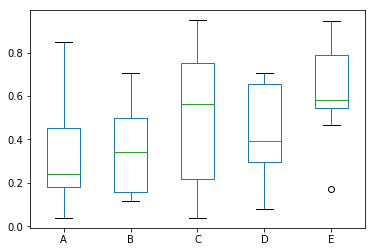六、块型图

可以使用Series.plot.area()或DataFrame.plot.area()方法创建区域图形。


import pandas as pd
import numpy as np

df = pd.DataFrame(np.random.rand(10, 4), columns=['a', 'b', 'c', 'd'])
df.plot.area()


Python

执行上面示例代码，得到以下结果 -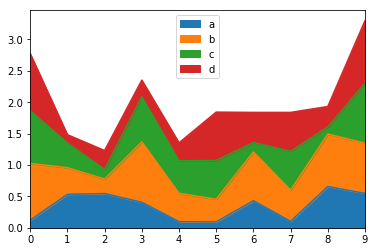七、散点图

可以使用DataFrame.plot.scatter()方法创建散点图。


import pandas as pd
import numpy as np
df = pd.DataFrame(np.random.rand(50, 4), columns=['a', 'b', 'c', 'd'])
df.plot.scatter(x='a', y='b')


Python

执行上面示例代码，得到以下结果 -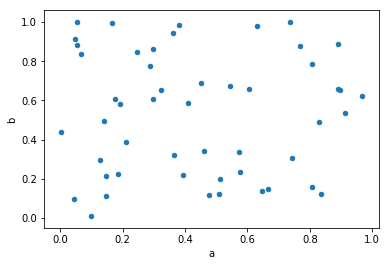八、饼状图

饼状图可以使用DataFrame.plot.pie()方法创建。


import pandas as pd
import numpy as np

df = pd.DataFrame(3 * np.random.rand(4), index=['a', 'b', 'c', 'd'], columns=['x'])
df.plot.pie(subplots=True)


Python

执行上面示例代码，得到以下结果 -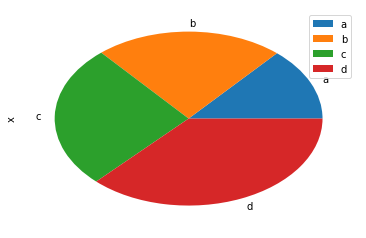参考文章：

 Pandas可视化

 Python for Data Analysis，Wes McKinney，public in 2012

展开全文数据分析 大数据
• ## Python--Pandas-数据可视化

万次阅读 多人点赞 2018-06-08 23:04:37
我们做数据可视化，其实就是就数据进行分析，使用Python做数据分析的，我想pandas必然是一个利器，一个非常强大的数据分析工具包，也集成了数据可视化的功能，一个集数据处理、分析、可视化于一身的工具，非常强大...
1.Pandas 简介

我们做数据可视化，其实就是就数据进行分析，使用Python做数据分析的，我想pandas必然是一个利器，一个非常强大的数据分析工具包，也集成了数据可视化的功能，一个集数据处理、分析、可视化于一身的工具，非常强大好用。pandas中的数据可视化已经可以满足我们大部分的要求了，也就省下了我们很多自己使用 如 matplotlib 来数据可视化的工作。

通常使用 pandas 进行下列的图形的快速绘图：

‘line’
‘bar’ or ‘barh’ for bar plots
‘hist’ for histogram
‘box’ for boxplot
‘area’ for area plots
‘scatter’ for scatter plots
‘pie’ for pie plots

对于本身熟悉 Matplotlib 的，就马上使用了，要不是不熟悉，也可通过下面的介绍快速上手。

2.数据集

在对数据进行可视化之前，我们先看一下数据集。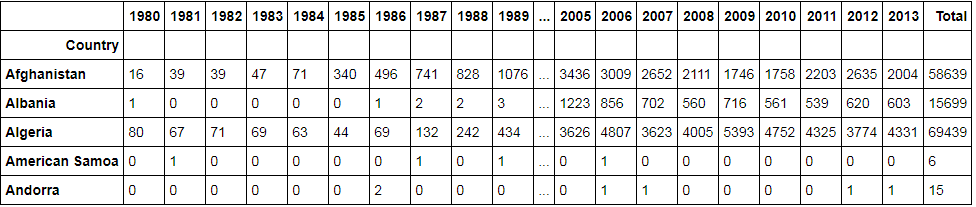3.可视化

3.1 画线

使用如下代码，绘画直线。

df.loc['Algeria'].plot(kind='line', label='Algeria') #取出 Algeria 这一行的数据
plt.legend(loc='upper left')一行代码，就对 index 为 ‘Algeria’ 数据画了线，这里我排除掉了 Total 这一列。

好像也不怎么样？看下面的代码及结果先：

df.T[['Albania', 'Algeria', 'Argentina']].plot(kind='line')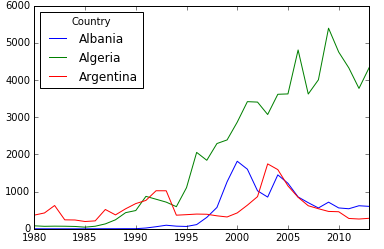很轻易的，我们就画了对应三条线，并且图例说明已经自动生成，已经看出它比 matplotlib 的方便的地方。这里有一个问题需要注意的是，我对数据集进行了转置，也就是行列互换。新的数据集如下：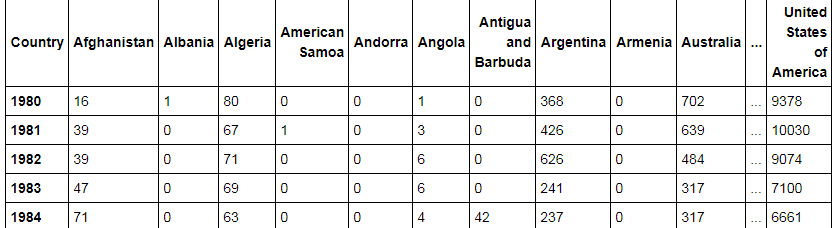在对具有多行的数据进行绘图时，pandas会将index作为X轴，对应列的数据作为Y轴，而对应列的Column则作为Line。意思就是给每个列画一条线。所以我们需要作出转置的操作。

3.2 直方图

观察下面代码：

count, bin_edges = np.histogram(df_can['2013'])
#将数据分成等间距的10个区间，count表示对应区间内有多个数据，bin_edges则是划分的区间，结果如下图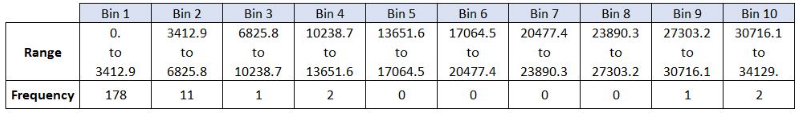df_can['2013'].plot(kind='hist', figsize=(8, 5), xticks=bin_edges)

plt.title('Histogram of Immigration from 195 countries in 2013') # add a title to the histogram
plt.ylabel('Number of Countries') # add y-label
plt.xlabel('Number of Immigrants') # add x-label

plt.show()

pandas 中绘画直方图，也是默认分成10个区间，跟np.histogram的划分一致，所以我们并不需要传入什么数据，只需确定绘画直方图，对于 xticks 可传可不传，若是没有传入xticks，则绘出的直方图的 X轴的区间则不那么直观。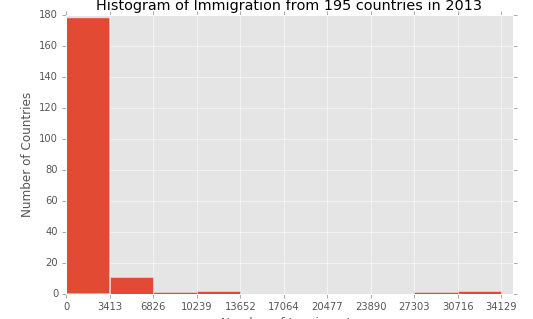像绘画条一样，我们也可以绘出若干个直方图。同样我们需要对数据进行转置。

df_t = df_can.loc[['Denmark', 'Norway', 'Sweden'], years].transpose()
df_t.head()

前5条数据如下：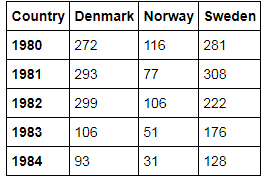df_t.plot(kind='hist', figsize=(10, 6))

plt.title('Histogram of Immigration from Denmark, Norway, and Sweden from 1980 - 2013')
plt.ylabel('Number of Years')
plt.xlabel('Number of Immigrants')

plt.show()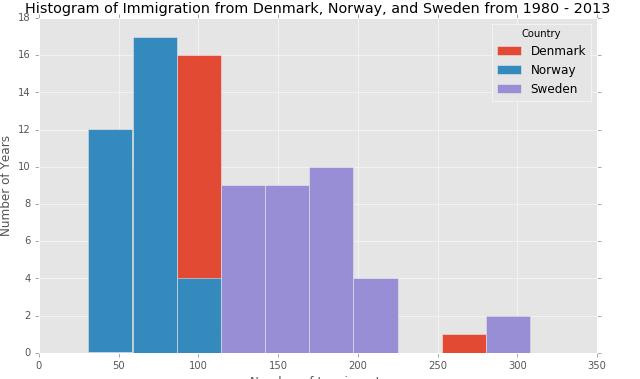可以发现的确是画出了三类直方图。可是有些类别的数据似乎覆盖掉了，我们观察不到，这不是好的例子，所以我们对Plot传入一些参数，使得被覆盖的数据还是可视的。

count, bin_edges = np.histogram(df_t, 15)

# un-stacked histogram
df_t.plot(kind ='hist',
figsize=(10, 6),
bins=15,
alpha=0.6,
xticks=bin_edges,
color=['coral', 'darkslateblue', 'mediumseagreen']
)

plt.title('Histogram of Immigration from Denmark, Norway, and Sweden from 1980 - 2013')
plt.ylabel('Number of Years')
plt.xlabel('Number of Immigrants')

plt.show()

上面我们修改了区间的个数，并且主要的是我们传入 alpha 透明度的参数，这就使得被覆盖的数据可视了。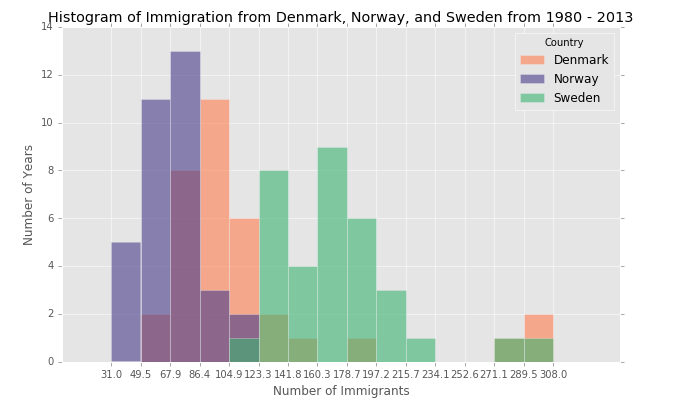还有下面一种修改

df_t.plot(kind ='hist',
figsize=(10, 6),
bins=15,
stacked=True,
xticks=bin_edges,
color=['coral', 'darkslateblue', 'mediumseagreen']
)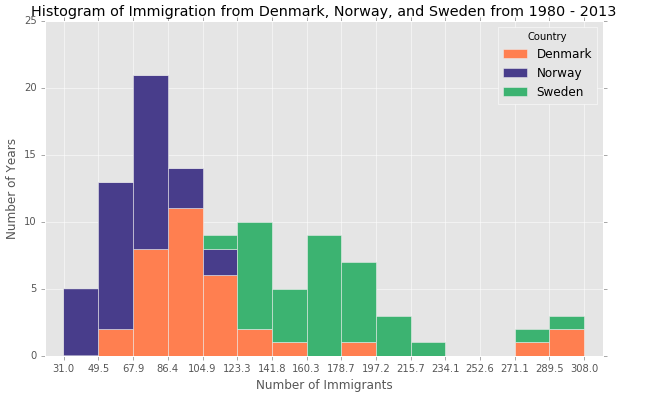3.3条形图

首先看一下我们将要进行可视化的数据

df_iceland = df_can.loc['Iceland', years]
df_iceland.head()

数据是冰岛1980-2013的移民数据，下面只展示前5条数据。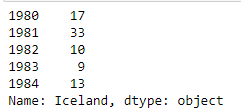绘画条线图很简答，代码如下：

# step 2: plot data
df_iceland.plot(kind='bar', figsize=(10, 6))
df_iceland.plot(kind='line')
plt.xlabel('Year') # add to x-label to the plot
plt.ylabel('Number of immigrants') # add y-label to the plot
plt.title('Icelandic immigrants to Canada from 1980 to 2013') # add title to the plot

plt.show()

条形图有分垂直，以及水平的，上面的就是垂直的。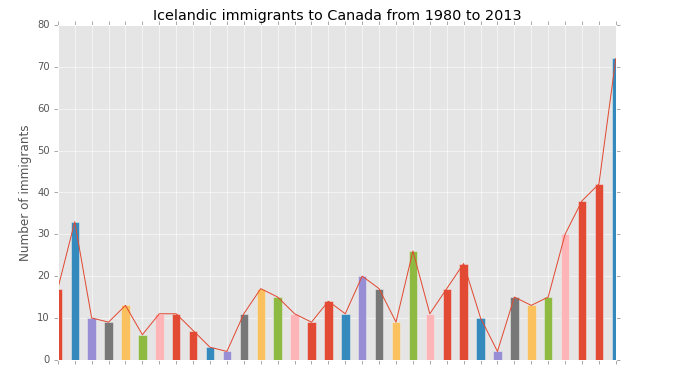df_iceland.plot(kind='barh', figsize=(10, 6))

只需要将 kind = ‘bar’ 换成 kind = ‘barh’ 就可以了。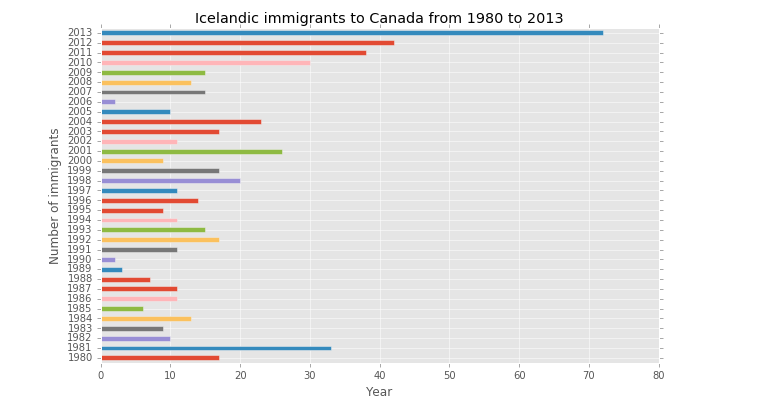3.4 饼图

首先观察一下将要来绘画饼图的数据;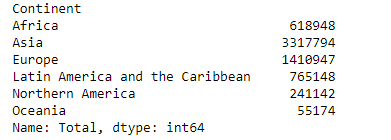通过一下代码绘画饼图：

# autopct create %, start angle represent starting point
df_continents['Total'].plot(kind='pie',
figsize=(5, 6),
startangle=90,     # start angle 90° (Africa)
)

plt.title('Immigration to Canada by Continent [1980 - 2013]')
plt.axis('equal') # Sets the pie chart to look like a circle.

plt.show()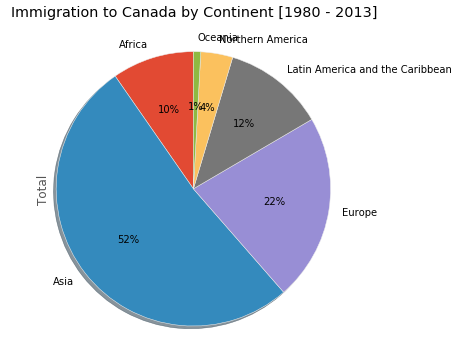可以发现一些label重叠的情况，为解决这种情况我们需要再传入一些参数：

colors_list = ['gold', 'yellowgreen', 'lightcoral', 'lightskyblue', 'lightgreen', 'pink']
explode_list = [0.1, 0, 0, 0, 0.1, 0.1] # ratio for each continent with which to offset each wedge.

df_continents['Total'].plot(kind='pie',
figsize=(15, 6),
autopct='%1.1f%%',
startangle=90,
labels=None,         # turn off labels on pie chart
pctdistance=1.12,    # the ratio between the center of each pie slice and the start of the text generated by autopct
explode=explode_list # 'explode' lowest 3 continents
)

# scale the title up by 12% to match pctdistance
plt.title('Immigration to Canada by Continent [1980 - 2013]', y=1.12)

plt.axis('equal')

plt.legend(labels=df_continents.index, loc='upper left')

plt.show()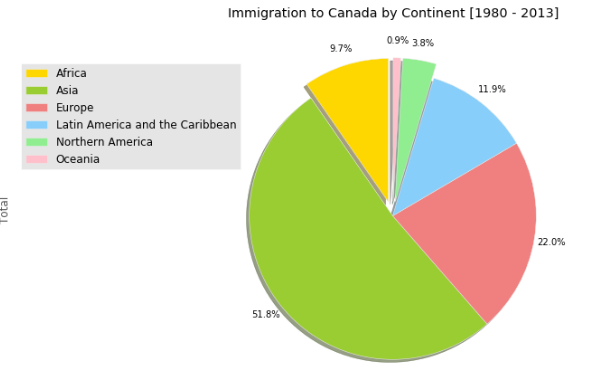3.5 Area

使用的数据如下：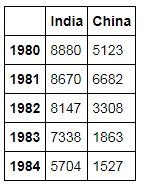fig, (ax1, ax2) = plt.subplots(2)
df_CI.plot(kind='area', stacked=False, ax=ax1)
df_CI.plot(kind='area', ax=ax2)

ax1 中就像是画 India 和 China 的线，然后进行填充，ax2中 stacked=True，数据的值就会叠加，叠加的方向是 DataFrame 从左向右。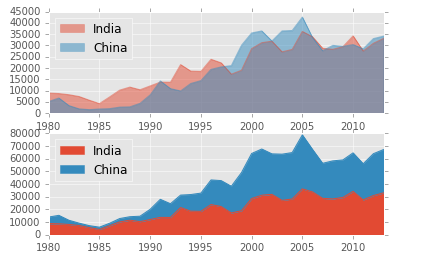3.6 Box 箱型图

数据概览如下：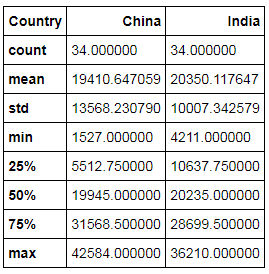df_CI.plot(kind='box')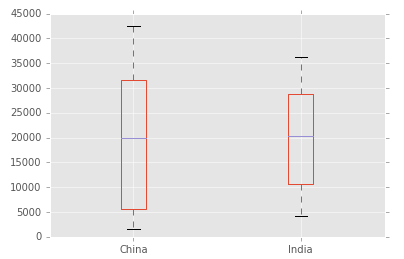3.7 Scatter 散点图

部分数据展示：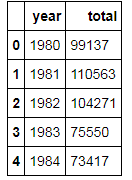df_tot.plot(kind='scatter', x='year', y='total', figsize=(10, 6), color='darkblue')

plt.title('Total Immigration to Canada from 1980 - 2013')
plt.xlabel('Year')
plt.ylabel('Number of Immigrants')

plt.show()

不同其他plot，散点图需要传入 x、y 的名，然后会自动在 df_tot 中寻找，跟 pyplot.plot() 中的类似。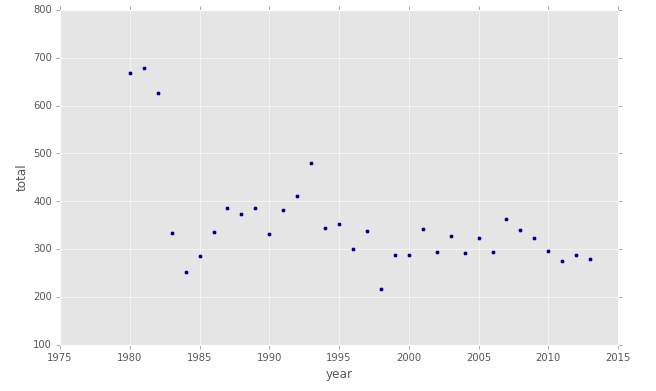展开全文Pandas
• 6款Python可视化工具，各有所长，适用于不同的场景，但是，它们都有一个共同点：强大且好用！下面就开始正文吧！ 前言 视觉是人们很重要的一种感官，所以，可视化，在数据相关的研发中可以起到“四两拨千斤”的...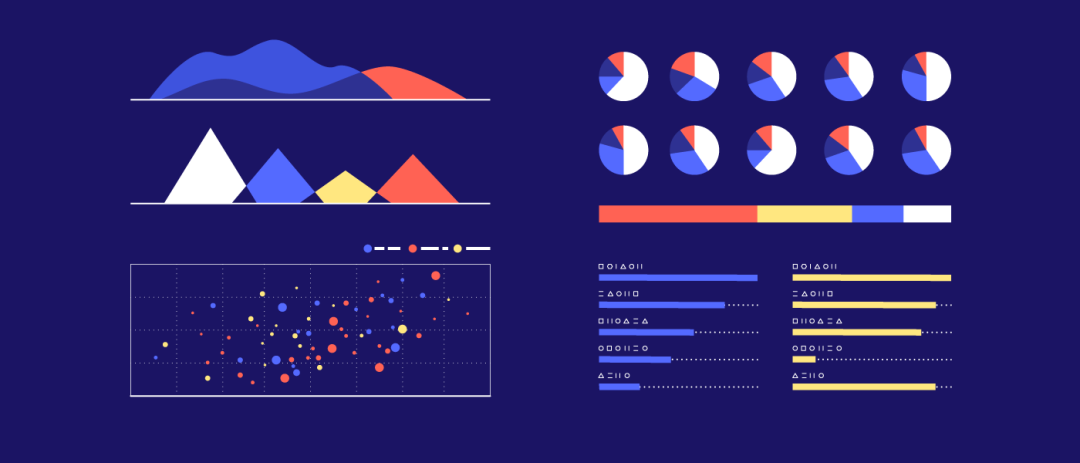6款Python可视化工具，各有所长，适用于不同的场景，但是，它们都有一个共同点：强大且好用！下面就开始正文吧！

前言

视觉是人们很重要的一种感官，所以，可视化，在数据相关的研发中可以起到“四两拨千斤”的作用。如果从直接查看离线存储在各类数据库中杂乱无章的数据，会让人瞬间感到崩溃、没有头绪。但是，如果对数据进行可视化，就变得一目了然。

例如，我们可以通过可视化看到一年中不同月份的降雨量、中国各个城市房价的对比、不同行业的薪资水平等。

Python相关的开发工作，很难绕过数据这一关，无论是做数据分析与挖掘，还是机器学习、计算机视觉。因此，一款好用的Python可视化工具，可以让开发效率得到极大的提升。本文就来介绍6款Python可视化工具，它们各有所长，适用于不同的场景，总会有一款适合你。

matplotlib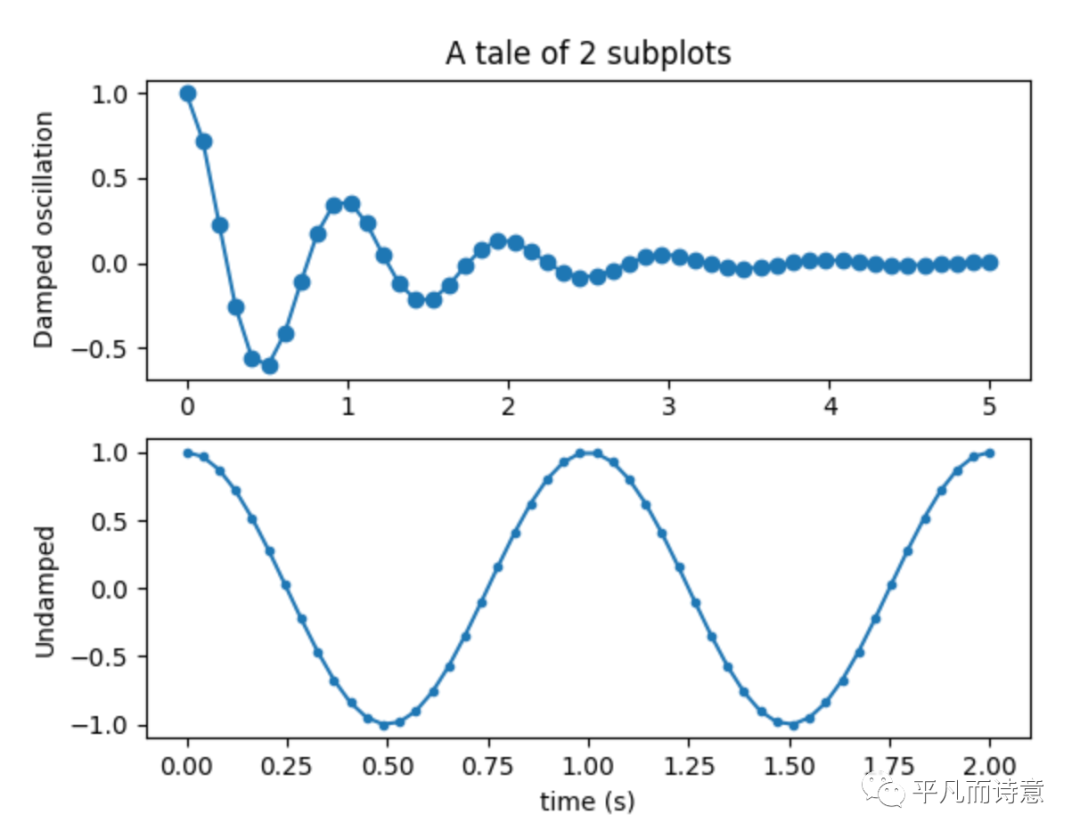再提到matplotlib就有一些老生常谈了，我想，凡是做过Python开发的，应该对matplotlib都不陌生，它俨然已经成了Python的标准库级别的第三方库。

matplotlib可以称得上中规中矩，它像大多数编程语言的标准库一样，读写、显示、子图、常见图形，可以满足大多数需求常见。

pyecharts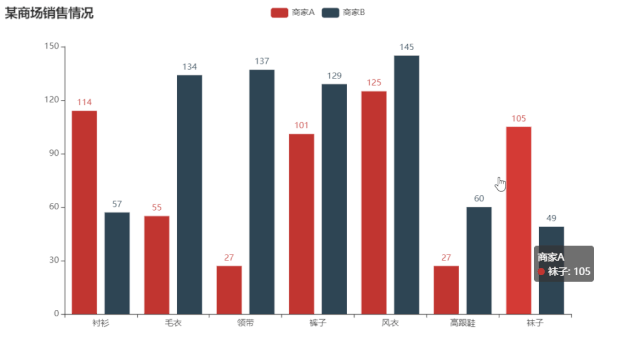pyecharts是基于百度开源的Echarts开发的Python版可视化工具。

如果说matplotlib中规中矩，那么pyecharts可以称得上强大，例如，

支持多达400+地图

支持Jupyter Notebook、JupyterLab

...

 

from pyecharts.charts import Bar
from pyecharts import options as opts
# V1 版本开始支持链式调用
bar = (
Bar()
.add_xaxis(["衬衫", "毛衣", "领带", "裤子", "风衣", "高跟鞋", "袜子"])
.add_yaxis("商家A", [114, 55, 27, 101, 125, 27, 105])
.add_yaxis("商家B", [57, 134, 137, 129, 145, 60, 49])
.set_global_opts(title_opts=opts.TitleOpts(title="某商场销售情况"))
)
bar.render()
# 不习惯链式调用的开发者依旧可以单独调用方法
bar = Bar()
bar.add_xaxis(["衬衫", "毛衣", "领带", "裤子", "风衣", "高跟鞋", "袜子"])
bar.add_yaxis("商家A", [114, 55, 27, 101, 125, 27, 105])
bar.add_yaxis("商家B", [57, 134, 137, 129, 145, 60, 49])
bar.set_global_opts(title_opts=opts.TitleOpts(title="某商场销售情况"))
bar.render()

更重要的是pyecharts可以保存为离线的HTML文件，能够将图标详细数据离线保存，便于离线分析。

plotly.py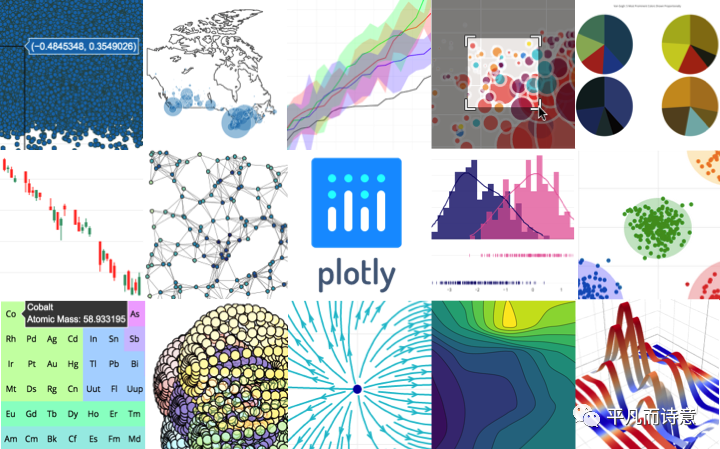相对于前两款Python可视化工具，plotly.py更偏重于交互式图形可视化。

plotly.py是基于plotly.js进行开发，它继承了plotly.js的诸多优点，例如，可以绘制科学图表，3D图形，统计图表，SVG地图，财务图表等丰富图形。

AutoViz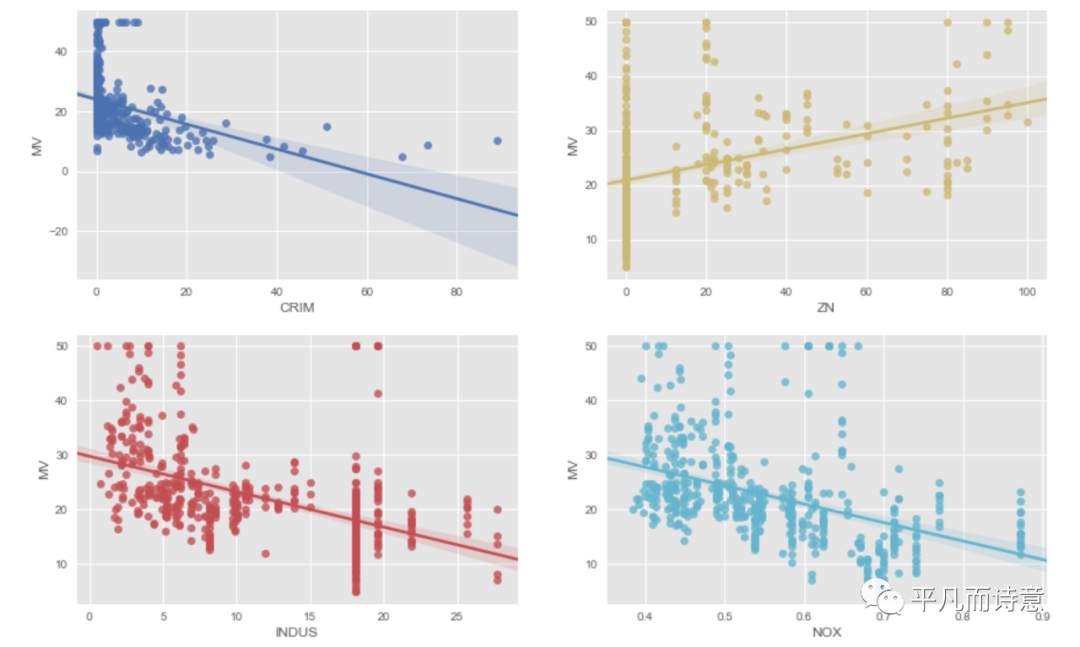数据可视化，大多数都需要把数据读取到内存中，然后对内存中的数据进行可视化。但是，对于真正令人头疼的是一次又一次的开发读取离线文件的数据接口。

而AutoViz就是用于解决这个痛点的，它真正的可以做到1行代码轻松实现可视化。它可以同时兼容txt、json、csv等主流离线数据格式，比较适合于机器学习、计算机视觉等涉及离线数据较多的应用场景。

Altair

Altair是一款基于Vega 和Vega-Lite开发的统计可视化库。

 

import altair as alt
from vega_datasets import data
source = data.unemployment_across_industries.url
alt.Chart(source).mark_area().encode(
alt.X('yearmonth(date):T',
axis=alt.Axis(format='%Y', domain=False, tickSize=0)
),
alt.Y('sum(count):Q', stack='center', axis=None),
alt.Color('series:N',
scale=alt.Scale(scheme='category20b')
)
).interactive()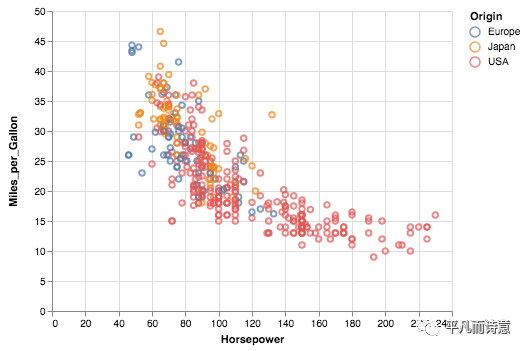Altair构建在强大的Vega-Lite JSON规范之上，并且具有API简单、友好、一致等诸多优点。因此，通过使用Altair，你可以把更多时间花费在理解数据和业务逻辑上，用最简短的代码实现数据可视化。

plotly前面已经介绍过，是一款非常强大的绘图工具。而pandas又是Python数据处理中非常常用的一个第三方库，那么，能不能把这两款强大的工具结合在一起呢？

 

df=cf.datagen.ohlc()
qf=cf.QuantFig(df,title='First Quant Figure',legend='top',name='GS')
qf.iplot()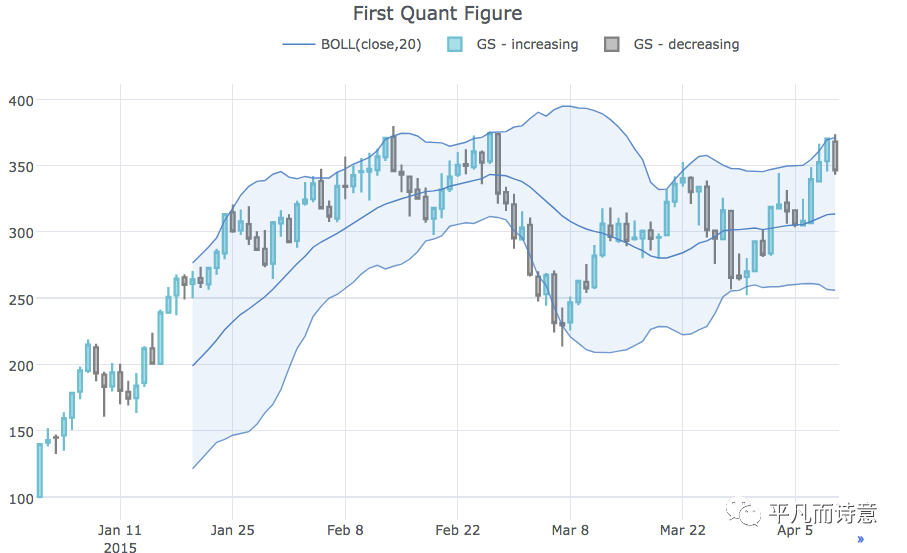结语

上述介绍的6款Python可视化工具，各有所长，特色明显：

matplotlib适用场景广泛、中规中矩。

AutoViz适合离线数据可视化。

plotly.py见长与交互可视化。

...

每位同学的工作场景不同，需求自然也不同，各位可以根据自己的的需求自行从中选择一款最适合自己的可视化工具！

睡前人工智能共享实验室新功能上线啦！

https://www.edu.futurelab.tv/

展开全文大数据
• 数据 链接 以下是本数据集的13个特征变量的详细说明： order_id：订单ID，数字组合而成，例如4283851335。 order_date：订单日期，格式为YYYY-MM-DD，例如2013-10-17。 order_time：订单日期，格式为HH:MM:SS，例如...
数据
链接
以下是本数据集的13个特征变量的详细说明：

order_id：订单ID，数字组合而成，例如4283851335。
order_date：订单日期，格式为YYYY-MM-DD，例如2013-10-17。
order_time：订单日期，格式为HH:MM:SS，例如12:54:44。
cat：商品一级类别，字符串型，包含中文、英文。
pro_id：商品ID，数字组合而成。
pro_brand：商品品牌，字符串型，包含中文、英文。
total_money：商品销售金额，浮点型。
total_quantity：商品销售数量，整数型。
order_source：订单来源，从哪个渠道形成的销售，字符串型，包含中文、英文。
pay_type：支付类型，字符串型，包含中文、英文。
use_id：用户ID，由数字和字母等组成的字符串。
city：用户订单时的城市，字符串型，中文。

数据清洗
# 模块导入
import pandas as pd
import numpy as np
import matplotlib.pyplot as plt
%matplotlib inline

# 数据载入
dtypes = {'order_id': np.object,
'pro_id': np.object,
'use_id': np.object}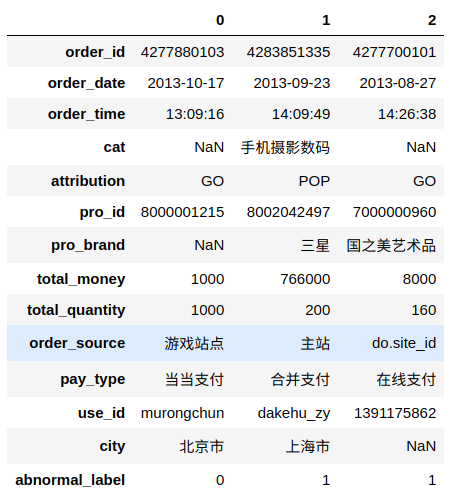# 数据行列
df.shape
#(134190, 12)

# 缺失值
df.isna().sum()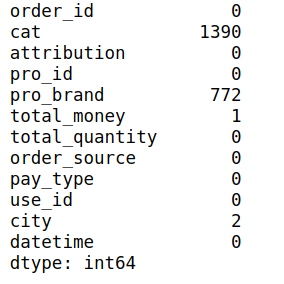不是很多直接舍弃:
# 弃去缺失值
df.dropna(inplace=True)

时间数据处理:
# 合并时间，并更改类型，弃去原来时间
df['datetime'] = (df.order_date + ' ' + df.order_time ).astype('datetime64[ns]')
df.drop(columns=['order_date', 'order_time', 'abnormal_label'], inplace=True)

# 设置时间段
start_time = pd.datetime(2013, 9, 1)
end_time = pd.datetime(2013, 10, 1)

# 根据时间段截取数据
df_time = df[(df.datetime < end_time) & (df['datetime'] > start_time)]

# 提取一个月中的每天成列
df_time['day'] = df_time.datetime.dt.day

# 获取各列包含种类
df.nunique()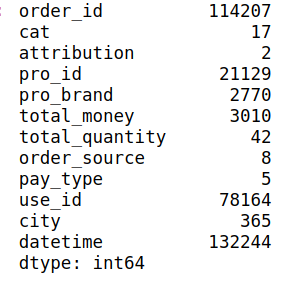可视化
plot.bar()
简单的柱状图
# 每天的订单量
df_time.day.value_counts().sort_index().plot.bar()
plt.savefig('bar1.png')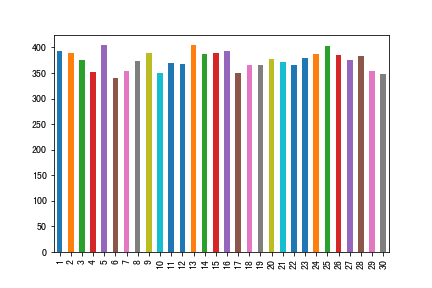添加其他设置:
# 一个月中每个类别商品的品牌数量 使用figsize更改画幅大小 title设置标题
df_time.pro_brand.groupby(df_time.cat).nunique().plot.bar(
figsize=(10,5),
title='商品品类品牌数量'
)
plt.savefig('bar2.png')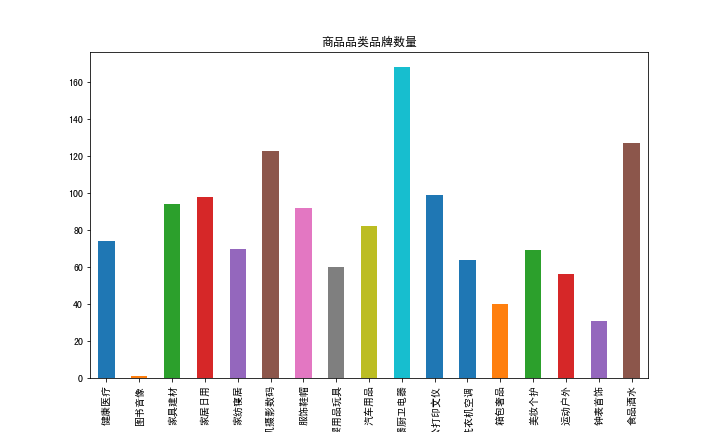# 一个月中每个类别商品用户数量 使用color更改颜色
df_time.use_id.groupby(df_time.cat).nunique().plot.bar(figsize=(10,5), color='red')
plt.title('商品品类买家数量')
plt.savefig('bar3.png')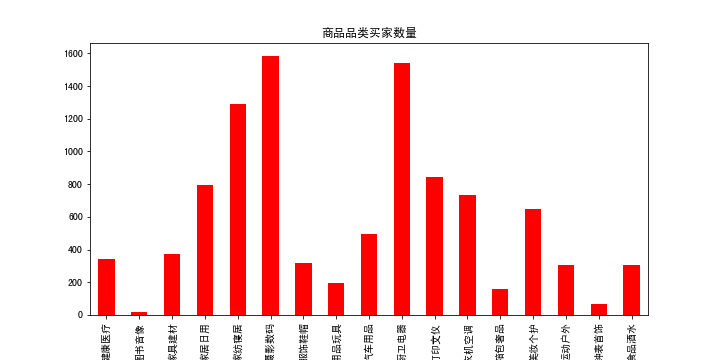更改字体大小:
# 一个月中每个类别商品商品数量 使用fontsize更改字体大小
df_time.pro_brand.groupby(df_time.cat).nunique().plot.bar(
figsize=(10,5),
color='g',
fontsize=14
)
plt.title('商品品类商品数量', fontsize=16)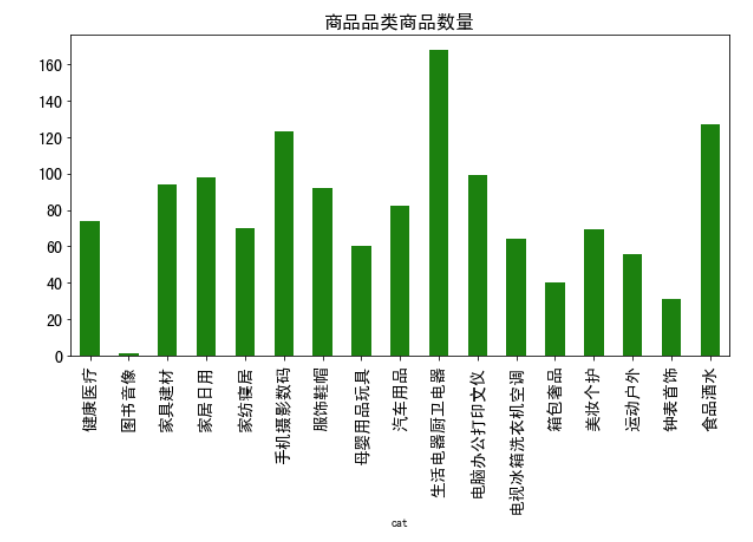堆叠:
# 每种类商品不同渠道获得订单量 使用stacked=True进行堆叠
df_time[['cat', 'order_source']].pivot_table(index=['cat'], columns=['order_source'], aggfunc=len, margins=False, fill_value=0).plot.bar(stacked=True)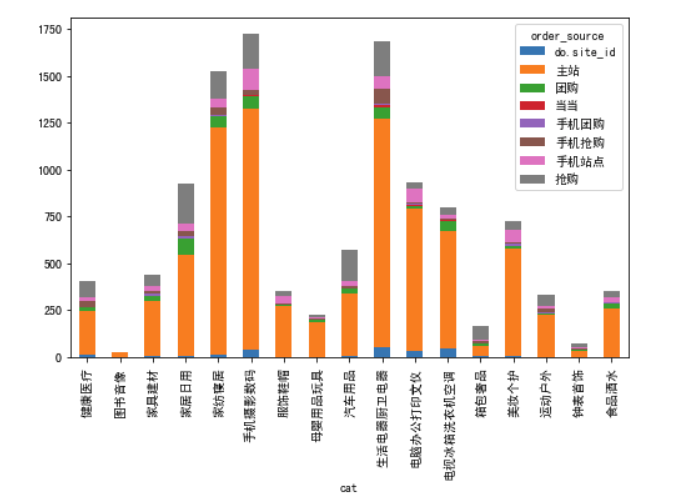# 每种商品以不同方式支付的订单量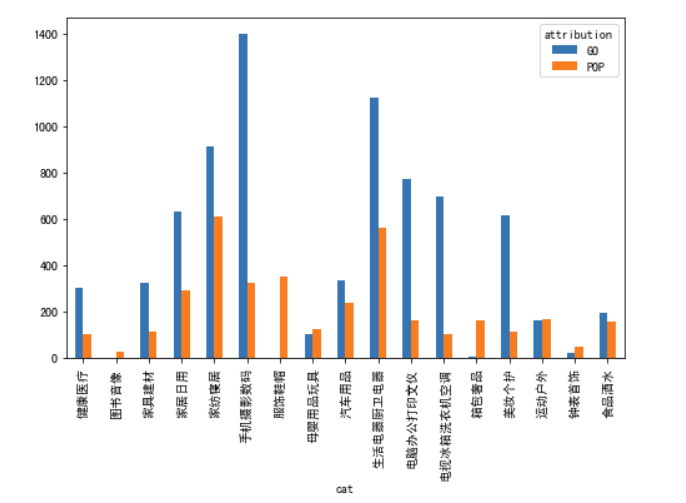plot.barh()
就是把柱状图横过来放
# 不同商品种类商品渠道来源情况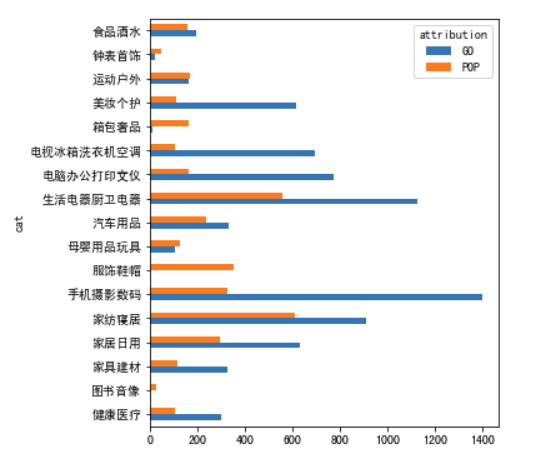plot.pie()
饼状图用于描述占比
# 售卖不同种类商品占比情况
df_time.cat.value_counts().plot.pie(figsize=(8,8))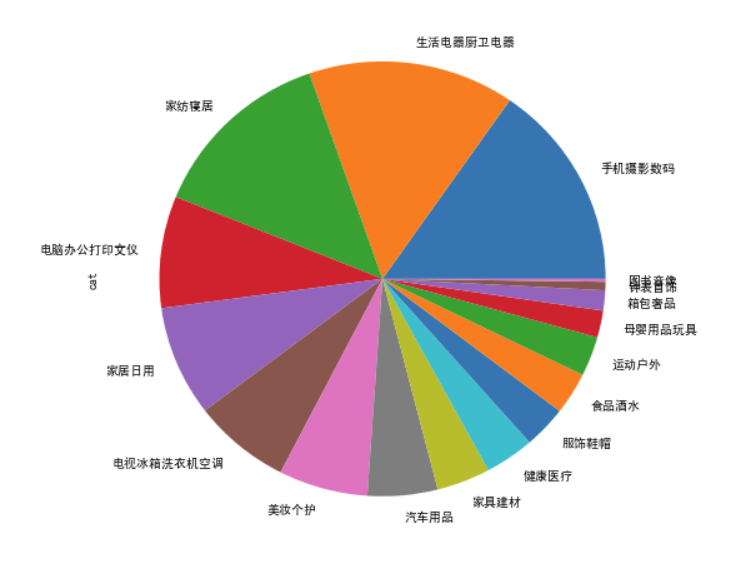plot.line()
折线图
# 一个月每天的成交金额走势
df_time.total_money.groupby(df_time.day).sum().plot.line()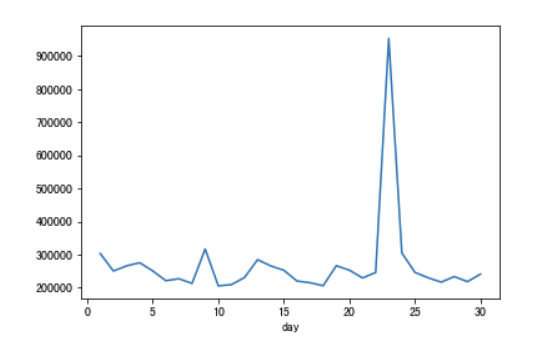# 一个月每天的成交金额, 成交商品量走势 使用subplots=True进行分图
pd.DataFrame(df_time.total_money.groupby(df_time.day).sum()).join(df_time.total_quantity.groupby(df_time.day).sum()).plot.line(subplots=True)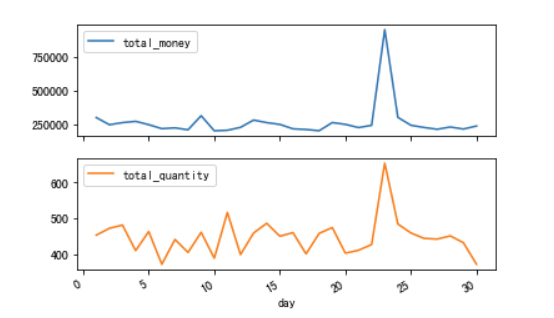plot.area()
面积填充图
# 一个月每天不同支付方式占比走势
pd.crosstab(df_time.day, df_time.pay_type, margins=False).plot.area()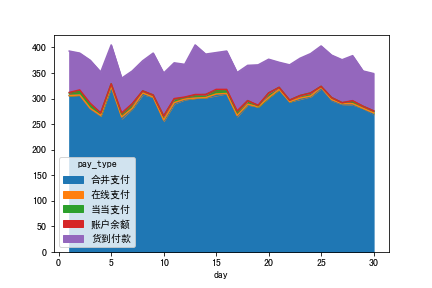plot.hist()
直方图:数据分布情况
# 每天成交金额直方图
df_time.total_money.groupby(df_time.day).sum().plot.hist()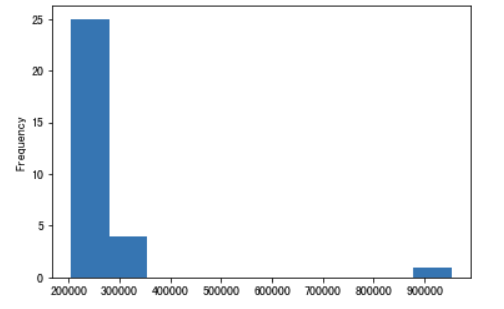plot.kde()
核密度估计
# 每天成交金额核密度估计
df_time.total_money.groupby(df_time.day).sum().plot.kde()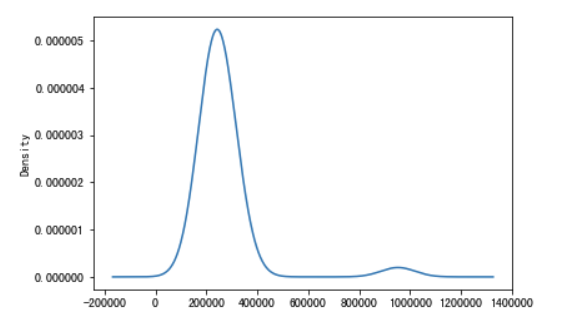plot.scatter()
散点图
# 成交总金额跟售卖量大体情况
df_time[(df_time.total_money < 100000) & (df_time.total_quantity < 40)].plot.scatter(x='total_quantity', y='total_money')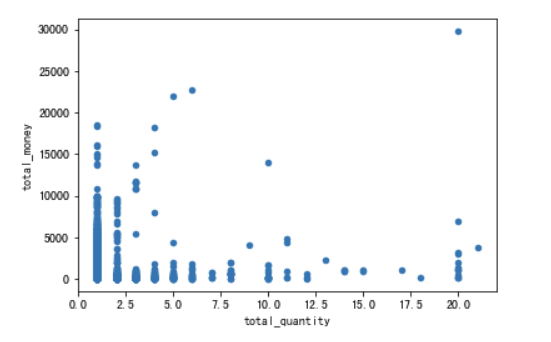plot.hexbin()
# 六边形分箱图 :密度通过颜色体现
df_time[(df_time.total_money < 100000)].plot.hexbin(x='total_quantity', y='total_money', gridsize=20, cmap="cool")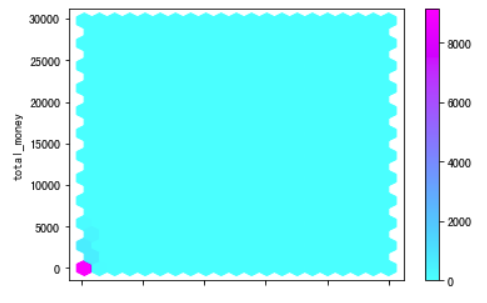plot.box()
箱线图:用于观察分布及异常值
# 成交金额箱线图
df_time[df_time.total_money <1000].total_money.plot.box()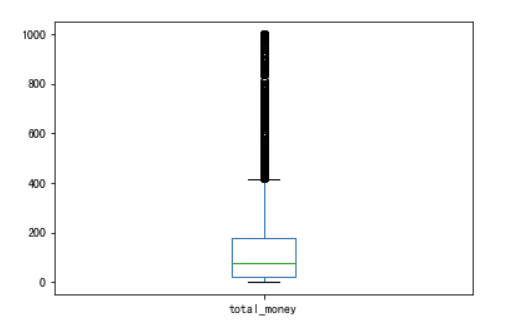boxplot()
箱线图:用于观察分布及异常值
# 不同支付方式成交金额箱线图
df_time[df_time.total_money <1000].boxplot(column=['total_money'], by='pay_type')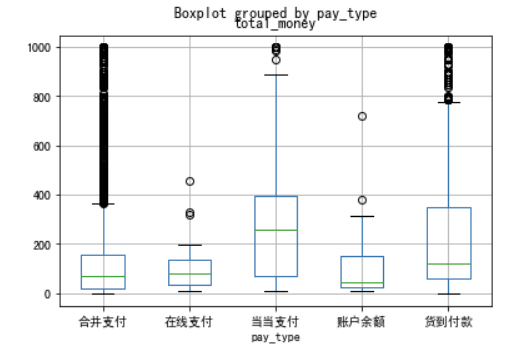展开全文pandas
• 6款Python可视化工具，各有所长，适用于不同的场景，但是，它们都有一个共同点：强大且好用！下面就开始正文吧！ http://www.makeru.com.cn/live/detail/1637.html?s=144282 前言 视觉是人们很重要的一种感官，所以...大数据 数据分析
• 数据可视化能够提取数据的结论见解和趋势。快速浏览Python 生态系统中的可视化工具，重点把Bokeh 作为渲染和展示大数据集的强劲工具。 Bokeh 是Python Anaconda 生态系统的一部分......spark Anaconda Bokeh
• ## python数据可视化

千次阅读 2019-03-26 17:16:26
简介 特征工程之前用excel做的，简单的还行，稍稍复杂就非常慢了。考虑换用python，相关资料整理了下，备查 01，pycharm绘图不显示问题 pandas中Series、...标题：Python可视化学习（2）：Matplotlib快...
• Python 天气情况数据分析及可视化 环境配置 Pycharm开发环境 python 版本 python3.7 Anconda 集成开发环境 第三方库导入 ## pip install 模块 清华大学镜像源 import requests from bs4 import BeautifulSoup ...数据分析 爬虫
• 文章来源：实战统计学作者：梁斌炜Pandas 是 Python 的核心数据分析支持库，提供了快速、灵活、明确的数据结构，旨在简单、直观地处理关系型、标记...
• Numpy 是一个开源的python科学基础库,包含： 强大的N维数组对象,广播功能，整合c/c++代码的工具，线性代数,傅里叶变化等功能; # 引入 numpy import numpy as np # 初始数组 a = np.array([1, 2, 3, 4, 5]) # 0 ...
• 转载自：Segment Fault 本文作为学习过程中对matplotlib一些常用知识点的整理，方便查找。 强烈推荐ipython 无论你工作在什么项目上，IPython都是值得推荐的。利用ipython --pylab，可以进入PyLab模式，已经导入...
• 它是一种特定类型的流程图，图中延伸的分支的宽度对应数据流量的大小，通常应用于能源、材料成分、金融等数据的可视化分析。 流程图的一种 由若干个三元素组成（节点，边，流量） 遵循守恒定律，无论怎么流动，开端...
• python可视化基础知识 import pandas as pd import numpy as np #实现同一个cell输出多个结果 from IPython.core.interactiveshell import InteractiveShell InteractiveShell.ast_node_interactivity = "all" 1....
• 使用python实现灰色关联分析及其可视化 在这里总结一下的数学建模常用的几种模型评估方法，这里讲的是使用python的Pandas库和高效的Numpy科学计算库实现。如果想看用Python实现的模型的灵敏度分析，可以看我上一篇...机器学习 数据挖掘 灰色关联分析
• Python 进行金融数据可视化 Python量化的关键是金融数据可视化，无论是传统的K线图，还是现在的策略分析，都需要大量的可视化图表。具体到编程代码，就是使用Python绘图模块库绘图，比如传统的Python绘图模块...
• 可视化总结 import matplotlib as mpl import matplotlib.pyplot as plt import warnings warnings.filterwarnings("ignore") # 忽略警告信息输出 # mpl.style.use('ggplot') # 为了画图中文可以正常显示 plt....
• 简介 大脑是人类目前所知的最复杂的...近年来，随着机器学习的兴起，医学数据与机器学习结合使用的情况越来越多，而要有效的使用好医学数据，其前提就是处理好这些数据，本文内容会重点介绍如何使用Python来处理...
• ## python之panda模块理解与学习

万次阅读 多人点赞 2018-07-09 16:39:12
Pandas是Python的一个大数据处理模块。Pandas使用一个二维的数据结构DataFrame来表示表格式的数据，相比较于Numpy，Pandas可以存储混合的数据结构，同时使用NaN来表示缺失的数据，而不用像Numpy一样要手工处理缺失的...数据结构 大数据 索引
• 数据可视化可以让我们很直观的发现数据中隐藏的规律，察觉到变量之间的互动关系，可以帮助我们更好的给他人解释现象，做到一图胜千文的说明效果。常见的数据可视化库有：matplotlib 是最常...
• 简单的使用pandas这个数据可视化库来进行数据展示import pandas as pdimport matplotlib.pyplot as plt# 获取csv文件unrate = pd.read_csv("ces.csv")# print(unrate)unrate["data"]= pd.to_...人工智能 pandas
...